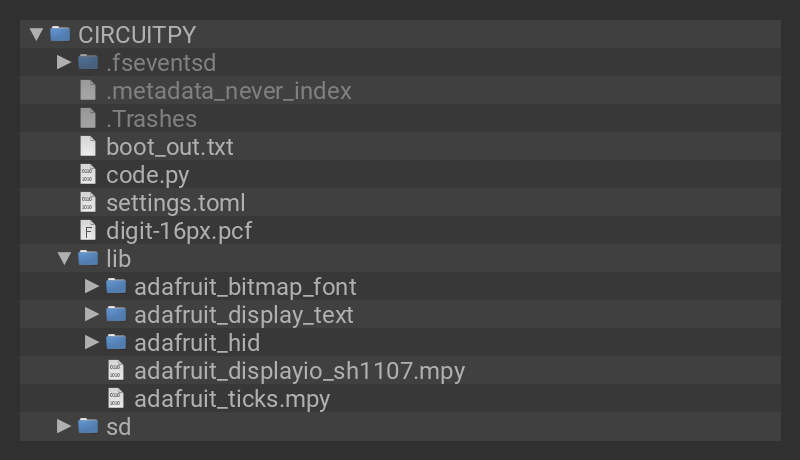Use CircuitPython 7.0.0-alpha.4 or newer for the code in this guide!

Are you new to using CircuitPython? No worries, there is a full getting started guide here.

Adafruit suggests using the Mu editor to edit your code and have an interactive REPL in CircuitPython. You can learn about Mu and installation in this tutorial.

Your project will use a specific set of CircuitPython libraries and the code.py file. In order to get the libraries you need, click on the Download Project Bundle link below, and uncompress the .zip file.

Drag the contents of the uncompressed bundle directory onto your board's CIRCUITPY drive, replacing any existing files or directories with the same names, and adding any new ones that are necessary.```# SPDX-FileCopyrightText: 2021 Jeff Epler for Adafruit Industries
#

import board
import displayio

try:
import usb_hid
except ImportError:
usb_hid = None

K_SQ = "√"
K_CL = "<clear>"
K_FN = "<fn>"
K_PA = "<paste>"

KEYMAP0 = [
K_CL, K_FN, '%',  '/',
'7',  '8',  '9',  '*',
'4',  '5',  '6',  '-',
'1',  '2',  '3',  '+',
'0',  '.',  K_SQ, '='
]

KEYMAP1 = [
K_CL, None, '', '',
'',   '',   '', '',
'',   '',   '', '',
'',   '',   '', '',
'',   '',   '', K_PA,
]

keymaps = {
0: KEYMAP0,
1: KEYMAP1,
}

# pylint: disable=redefined-outer-name
def lookup(layer, key_number):
while layer >= 0:
key = keymaps[layer][key_number]
if key is not None:
return key
layer -= 1
return None

displayio.release_displays()
# oled_reset = board.D9

# Use for I2C
i2c = board.I2C()  # uses board.SCL and board.SDA
# i2c = board.STEMMA_I2C()  # For using the built-in STEMMA QT connector on a microcontroller

# SH1107 is vertically oriented 64x128
WIDTH = 128
HEIGHT = 64

display = adafruit_displayio_sh1107.SH1107(display_bus, width=WIDTH, height=HEIGHT, rotation=180)
display.auto_refresh = False

text_area = label.Label(font, text=" ", line_spacing=0.95)
text_area.y = 8
display.show(text_area)

N = float

unary = {
K_SQ: lambda a: a**.5,
}

binary = {
'+': (lambda a, b: a+b, lambda a, b: a * (1+b/100)),
'-': (lambda a, b: a-b, lambda a, b: a * (1-b/100)),
'*': (lambda a, b: a*b, lambda a, b: a * (b/100)),
'/': (lambda a, b: a/b, lambda a, b: a / (b/100)),
}

class Calculator:
def __init__(self):
self._number1 = N("0")
self._number2 = None
self.entry = ""
self.op = None
self.keyboard = None
self.keyboard_layout = None

def paste(self, text):
if self.keyboard is None:
if usb_hid:
self.keyboard = Keyboard(usb_hid.devices)
self.keyboard_layout = KeyboardLayoutUS(self.keyboard)
else:
return

if self.keyboard_layout is None:
else:
text = str(text)
self.keyboard_layout.write(text)

self.trail = self.trail[-3:] + [str(msg).upper()]

@property
def number1(self):
return self._number1

@number1.setter
def number1(self, number):
self._number1 = number

@property
def number2(self):
if self.entry == "":
if self._number2 is not None:
return self._number2
return None
return N(self.entry)

@number2.setter
def number2(self, number):
self._number2 = number
self.entry = ''

def clear(self):
self.number1 = N("0")

def clear_entry(self):
self.number2 = None

def key_pressed(self, k): # pylint: disable=too-many-branches
if k == K_CL:
if self.entry:
self.entry = self.entry[:-1]
elif self.op:
print("clear op")
self.op = None
elif self.number2 is None:
self.clear()
else:
print("clear entry - op = ", self.op)
self.clear_entry()

if len(k) == 1 and k in "0123456789":
self.entry = self.entry + k

if k == "." and not "." in self.entry:
if self.entry == "":
self.entry = "0"
self.entry = self.entry + k

if k == K_PA:
if self.number2 is not None:
self.paste(self.number2)
else:
self.paste(self.number1)

if k == "=":
self.do_binary_op(0)

if k == "%":
self.do_binary_op(1)

op = unary.get(k)
if op:
self.do_unary_op(op)

if k in binary:
if self.number2 is not None:
if self.op:
self.do_binary_op(0)
else:
self.number1 = self.number2
self.clear_entry()
self.op = k

def do_unary_op(self, op):
if self.number2 is not None:
self.number2 = op(self.number2)
else:
self.number1 = op(self.number1)

def do_binary_op(self, i):
if self.op and self.number2 is not None:
op = binary[self.op][i]
self.op = None
self.number1 = op(self.number1, self.number2)
self.clear_entry()

def show(self):
rows = [""] * 4
trail = self.trail
if len(trail) > 0:
rows = trail[-1]
if len(trail) > 1:
rows = trail[-2]
if len(trail) > 2:
rows = trail[-3]

entry_or_number = self.entry or self.number2
cursor = ' :' if (self.number2 is None or self.entry != "") else ""
op = self.op or ''
op = 'd' if op == '/' else op
rows[-1] = f"{op}{entry_or_number or ''}{cursor}"
for r in rows:
print(r)
text_area.text = "\n".join(rows)

row_pins=(board.A2, board.A1, board.A3, board.A0, board.D0),
column_pins=(board.D25, board.D11, board.D12, board.D24))

calculator=Calculator()
calculator.show()

layer = 0
while True:
ev = km.events.get()
if ev:
key = lookup(layer, ev.key_number)
if ev.pressed:
if key == K_FN:
layer = 1
try:
calculator.key_pressed(key)
except Exception as e: # pylint: disable=broad-except
calculator.show()

elif ev.released:
if key == K_FN:
layer = 0

else:
display.refresh()
```

```km=keypad.KeyMatrix(